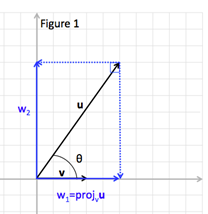# Decomposing a Vector into Components

In many applications it is necessary to decompose a vector into the sum of two perpendicular vector components. This is true of many physics applications involving force, work and other vector quantities. Perpendicular vectors have a dot product of zero and are called orthogonal vectors.

Figure 1 shows vectors u and v with vector u decomposed into orthogonal components w1 and w2.

Vector u can now be written u = w1 + w2, where w1 is parallel to vector v and w1 is perpendicular/orthogonal to w2. The vector component w1 is also called the projection of vector u onto vector v, projv u.The projv u can be calculated as follows:

PROJECTION OF U ONTO V:

Let u and v be nonzero vectors:

Once the vector component of projv uis found, since u = w1 + w2, component vector w2 can be found by subtracting w1 from u.

w2 = u - w1

Let's look at some examples.

To work these examples requires the use of various vector rules. If you are not familiar with a rule go to the associated topic for a review.

Example 1: Let $u=〈-2,2〉$ and $v=〈3,5〉$. Write vector u as the sum of two orthogonal vectors one of which is a projection of u onto v.
 Step 1: Find the projv u. $pro{j}_{v}u=\left[\frac{u·v}{\parallel v{\parallel }^{2}}\right]v={w}_{1}$ $pro{j}_{v}u=\left[\frac{u·v}{\parallel v{\parallel }^{2}}\right]v$ $pro{j}_{v}u=\left[\frac{\left(-2·3\right)+\left(2·5\right)}{{\sqrt{{3}^{2}+{5}^{2}}}^{2}}\right]〈3,5〉$ $pro{j}_{v}u=\left[\frac{-6+10}{{\sqrt{34}}^{2}}\right]〈3,5〉$ $pro{j}_{v}u=\left[\frac{4}{34}\right]〈3,5〉=\left[\frac{2}{17}\right]〈3,5〉$ $pro{j}_{v}u={〈}\frac{6}{17}{,}\frac{10}{17}{〉}$ Step 2: Find the orthogonal component. w2 = u - w1 w2 = u - w1 ${w}_{2}=〈-2,2〉-〈\frac{6}{17},\frac{10}{17}〉$ ${w}_{2}={〈}{-}\frac{40}{17}{,}\frac{24}{17}{〉}$ Step 3: Write the vector as the sum of two orthogonal vectors. u = w1 + w2 u = w1 + w2 $u={〈}\frac{6}{17}{,}\frac{10}{17}{〉}{+}{〈}{-}\frac{40}{17}{,}\frac{24}{17}{〉}$
Example 2: Given vector $u=〈1,3〉$ and $v=〈-4,5〉$, write u as a sum of two orthogonal vectors, one which is a projection of u onto v.
 Step 1: Find the projv u. $pro{j}_{v}u=\left[\frac{u·v}{\parallel v{\parallel }^{2}}\right]v={w}_{1}$ $pro{j}_{v}u=\left[\frac{u·v}{\parallel v{\parallel }^{2}}\right]v$ $pro{j}_{v}u=\left[\frac{\left(1·-4\right)+\left(3·5\right)}{{\sqrt{{\left(-4\right)}^{2}+{5}^{2}}}^{2}}\right]〈-4,5〉$ $pro{j}_{v}u=\left[\frac{-4+15}{{\sqrt{41}}^{2}}\right]〈-4,5〉$ $pro{j}_{v}u=\left[\frac{11}{41}\right]〈-4,5〉$ $pro{j}_{v}u={〈}{-}\frac{44}{41}{,}\frac{55}{41}{〉}$ Step 2: Find the orthogonal component. w2 = u - w1 w2 = u - w1 ${w}_{2}=〈1,3〉-〈-\frac{44}{41},\frac{55}{41}〉$ ${w}_{2}={〈}\frac{85}{41}{,}\frac{68}{41}{〉}$ Step 3: Write the vector as the sum of two orthogonal vectors. u = w1 + w2 u = w1 + w2 $u={〈}{-}\frac{44}{41}{,}\frac{55}{41}{〉}{+}{〈}\frac{85}{41}{,}\frac{68}{41}{〉}$

 Related Links: Math algebra The First Derivative Rule The Second Derivative Rule Pre Calculus

To link to this Decomposing a Vector into Components page, copy the following code to your site: